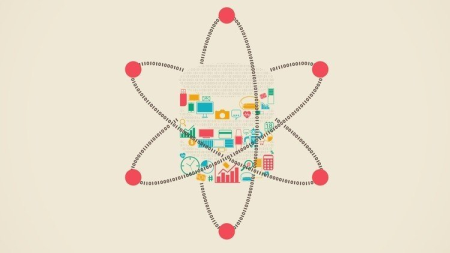MP4 | Video: h264, 1280×720 | Audio: AAC, 44.1 KHz, 2 Ch
Genre: eLearning | Language: English + .srt | Duration: 202 lectures (17h 3m) | Size: 6.96 GB

Machine learning with many practical examples.

Regression, Classification and much more

Create machine learning applications in Python as well as R

Apply Machine Learning to own data

You will learn Machine Learning clearly and concisely

Learn with real data: Many practical examples (spam filter, is fungus edible or poisonous etc. …)

No dry mathematics – everything explained vividly

Use popular tools like Sklearn, and Caret

You will know when to use which machine learning model

You should have programmed a little before.

No knowledge of Python or R is required.

All necessary tools (R, RStudio, Anaconda, …) will be installed together in the course.

This course contains over 200 lessons, quizzes, practical examples, … – the easiest way if you want to learn Machine Learning.

Step by step I teach you machine learning. In each section you will learn a new topic – first the idea / intuition behind it, and then the code in both Python and R.

Machine Learning is only really fun when you evaluate real data. That’s why you analyze a lot of practical examples in this course:

Estimate the value of used cars

Write a spam filter

Diagnose breast cancer

All code examples are shown in both programming languages – so you can choose whether you want to see the course in Python, R, or in both languages!

After the course you can apply Machine Learning to your own data and make informed decisions:

You know when which models might come into question and how to compare them. You can analyze which columns are needed, whether additional data is needed, and know which data needs to be prepared in advance.

This course covers the important topics:

Regression

Classification

On all these topics you will learn about different algorithms. The ideas behind them are simply explained – not dry mathematical formulas, but vivid graphical explanations.

We use common tools (Sklearn, NLTK, caret, data.table, …), which are also used for real machine learning projects.

What do you learn?

Regression:

Linear Regression

Polynomial Regression

Classification:

Logistic Regression

Naive Bayes

Decision trees

Random Forest

You will also learn how to use Machine Learning:

With complete practical example, explained step by step

Find the best hyper parameters for your model

"Parameter Tuning"

Compare models with each other:

How the accuracy value of a model can mislead you and what you can do about it

K-Fold Cross Validation

Coefficient of deteation

My goal with this course is to offer you the ideal entry into the world of machine learning.

Developers interested in Machine Learning